Secondary reactions and diffusion of tar during single coal particle pyrolysis
ZHANG Shengcheng , HE RongKey Laboratory for Thermal Science and Power Engineering of Ministry of Education, Department of Thermal Engineering, Tsinghua University, Beijing 100084, China
Abstract:A numerical model was developed to investigate the tar production during pyrolysis of a single coal particle. The model was based on the fragmentation and diffusion (FD) coal pyrolysis model and considered the secondary reactions and diffusion inside the coal particle. The model was validated by pyrolysis experiments in a drop-tube furnace and then used to investigate the influences of the final temperature, heating rate and particle diameter. The results show that the competition between the secondary reactions and tar diffusion greatly influences the tar production. Increases of the final temperature and the heating rate improve both the tar secondary reactions and the diffusion while increasing the particle diameter improves the tar secondary reactions and hinders the diffusion. The final tar yield decreases with increasing final temperature, increasing heating rate and increasing particle diameter.
Key words: coal     pyrolysis     tar     secondary reactions     diffusion

1 模型建立 1.1 热解模型

FD煤热解模型假设煤分子由2 500个单体通过共价键连接形成。每个单体由芳香簇和16种官能团组成。这16种官能团包括分解生成CO2的羧基、 分解生成CO的醚基、 分解生成CH4的甲基等。热解时,连接单体的共价键随机断裂,煤分子裂解为分子碎片,分子碎片中的共价键继续随机断裂形成更小的碎片,直到分子碎片足够小,成为焦油碎片或者气体碎片。当分子碎片只包含一个单体时,其为气体碎片,其中的16种官能团在随后的反应中分解形成轻质气体。当分子碎片包含的单体数目多于1个且碎片的相对分子质量小于阈值时,分子碎片为焦油碎片,在接下来的反应中裂解生成一次焦油。一次焦油或者在颗粒内部发生二次反应而消耗掉,或者扩散到颗粒外部而成为最终的产物。

 ${{M}_{r,th}}=\frac{a{{M}_{r,m}}+b}{{{T}_{p}}}.$ (1)

 \begin{align} & {{M}_{r,th}}=1723+774.3{{w}_{c}}-1411.1w_{c}^{2}-46327.3{{w}_{H}}+528920w_{H}^{2}-891.6{{w}_{o}} \\ & +326.8w_{o}^{2}+191.68{{w}_{vm}}-379.06w_{vm}^{2}. \\ \end{align} (2)

 ${{M}_{r,th}}=\frac{1800{{M}_{r,m}}+810000}{{{T}_{p}}}.$ (3)

FD煤热解模型的分子碎片生成反应、各种一次反应和二次反应的反应速率都遵循Arrhenius定律。

1.2 颗粒内部的扩散

 $\frac{\partial c}{\partial t}=D\frac{\partial }{\partial x}\left[ \left( 1+\delta \ln \frac{{{c}_{s}}}{c} \right)\frac{\partial c}{\partial x} \right].$ (4)

 $\delta ={{\left( 1-\theta \right)}^{4/3}}{{\left( \frac{{{r}_{m}}}{\lambda } \right)}^{1.25}}\exp \left[ 4.75+0.38{{f}_{d}}-3.27{{\left( \frac{{{r}_{m}}}{\lambda } \right)}^{0.41}} \right].$ (5)

 $D=\left\{ \begin{matrix} \frac{v}{3}{{d}_{m}}\theta \exp \left( .39-2.42{{f}_{d}} \right),0<\frac{{{d}_{m}}}{\lambda }\le 0.5; \\ \frac{v}{3}d_{m}^{0.0033\frac{{{d}_{m}}}{\lambda }+0.57}{{\theta }^{2.97-0.17\frac{{{d}_{m}}}{\lambda }}}{{e}^{-0.75-0.054{{f}_{d}}\frac{{{d}_{m}}}{\lambda }}},0.5<\frac{{{d}_{m}}}{\lambda }\le 10. \\ \end{matrix} \right.$ (6)

 ${{D}_{tar}}=1.86\times {{10}^{-22}}\frac{{{T}^{1.5}}}{P\sigma _{g,t}^{2}{{\Omega }_{D}}}{{\left\{ \frac{1}{325}+\frac{1}{20} \right\}}^{0.5}},$ (7)
 ${{\sigma }_{g,t}}=\frac{1}{2}\left( 3.4\times {{10}^{-10}}+{{10}^{-9}} \right),$ (8)
 ${{\Omega }_{D}}=3.538\times {{10}^{-3}}T+0.193{{e}^{-1.589\times {{10}^{-3}}T}}.$ (9)
1.3 实验与模型验证

 煤种 φ θ s/(107m2·m－3) fd 杨村 0.531 0.343 0.997 0.921 淮南 0.472 0.557 1.212 0.709 洛阳 0.2 0.292 1.03 0.768 永成 0.189 0.477 0.938 0.735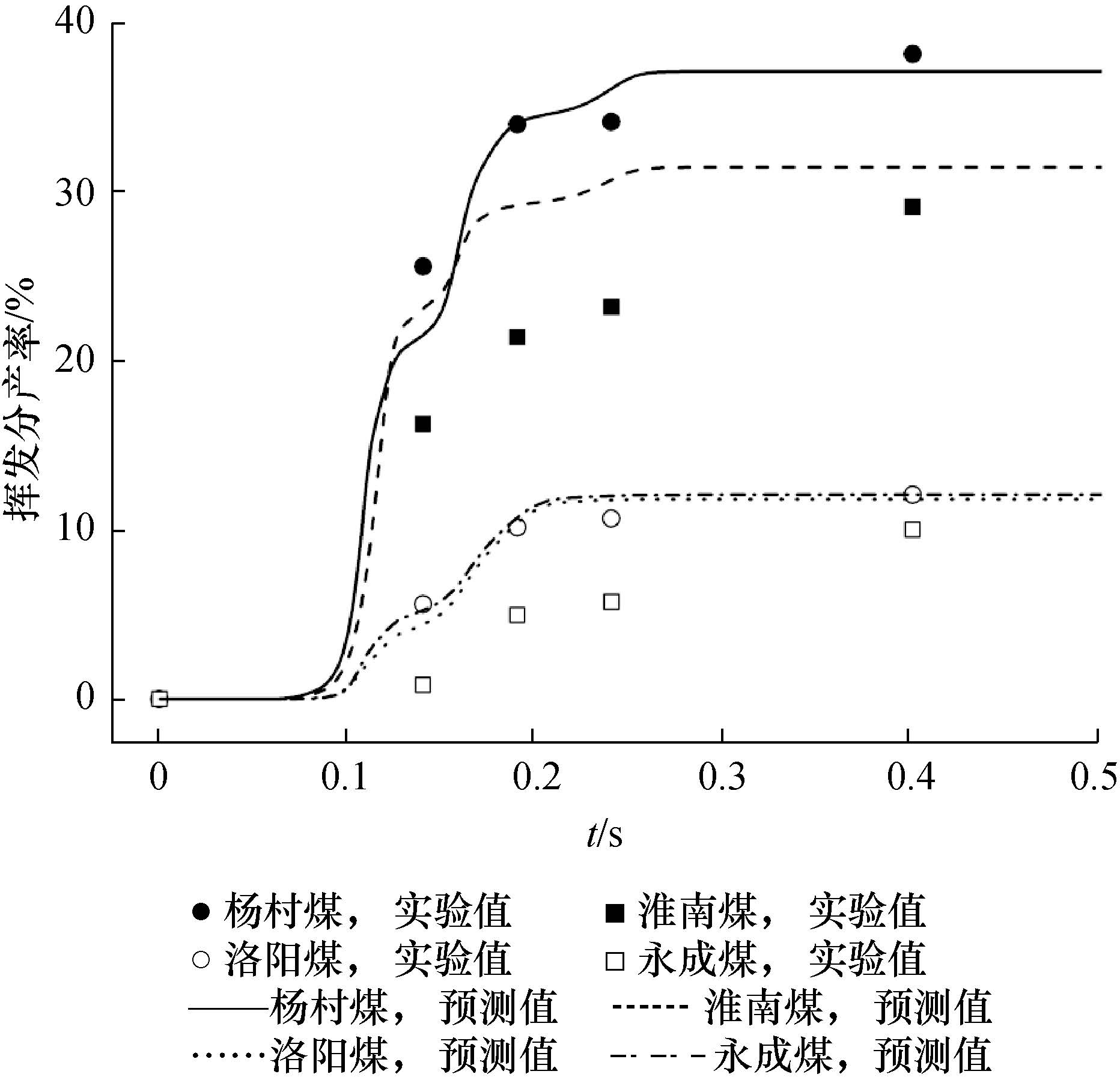图 1 实验测量的和模型预测的挥发分产率

2 焦油生成过程中二次反应与扩散的竞争

2.1 热解终温对焦油生成的影响图 2 一次焦油产率和热解终温的关系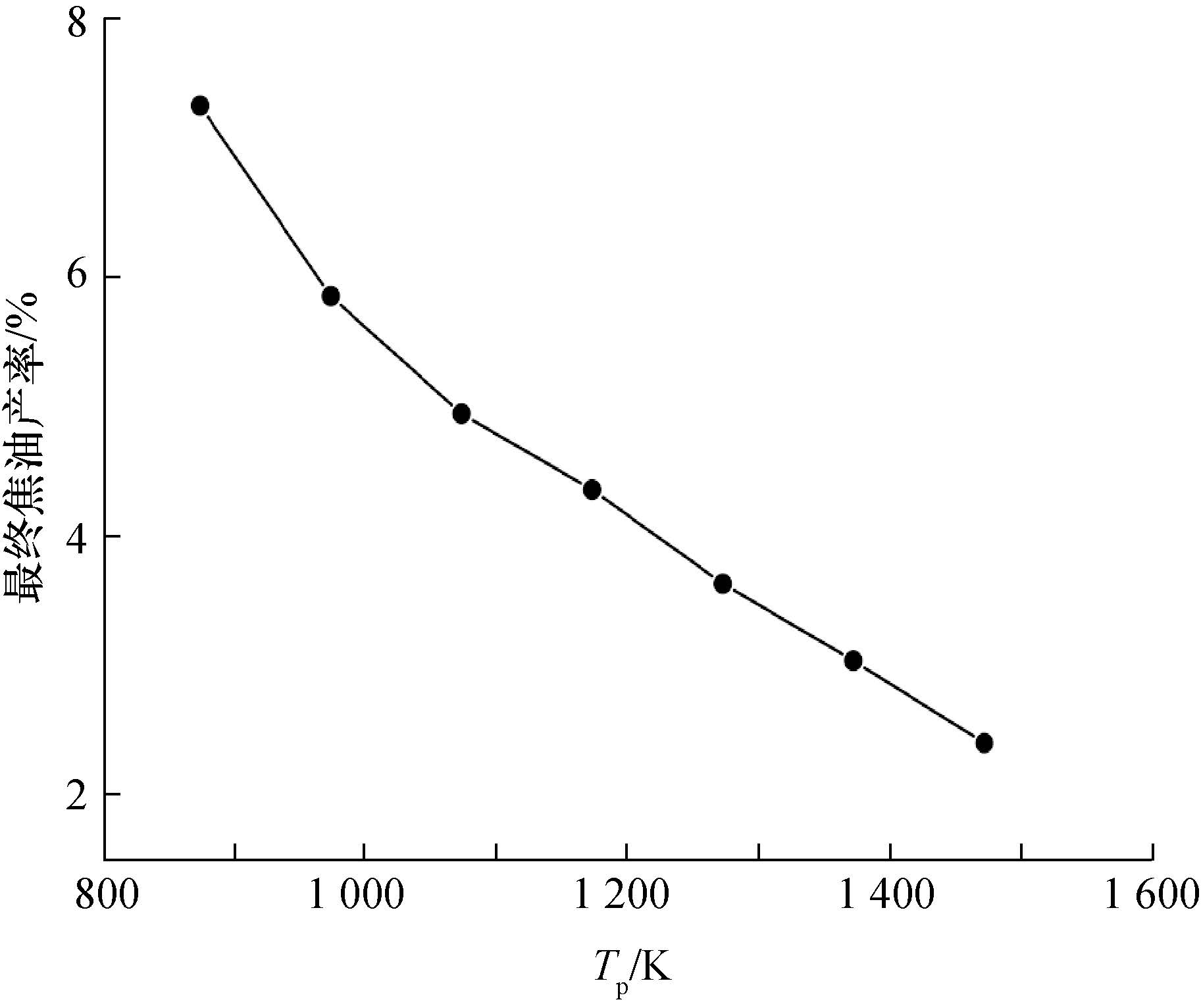图 3 最终焦油产率和热解终温的关系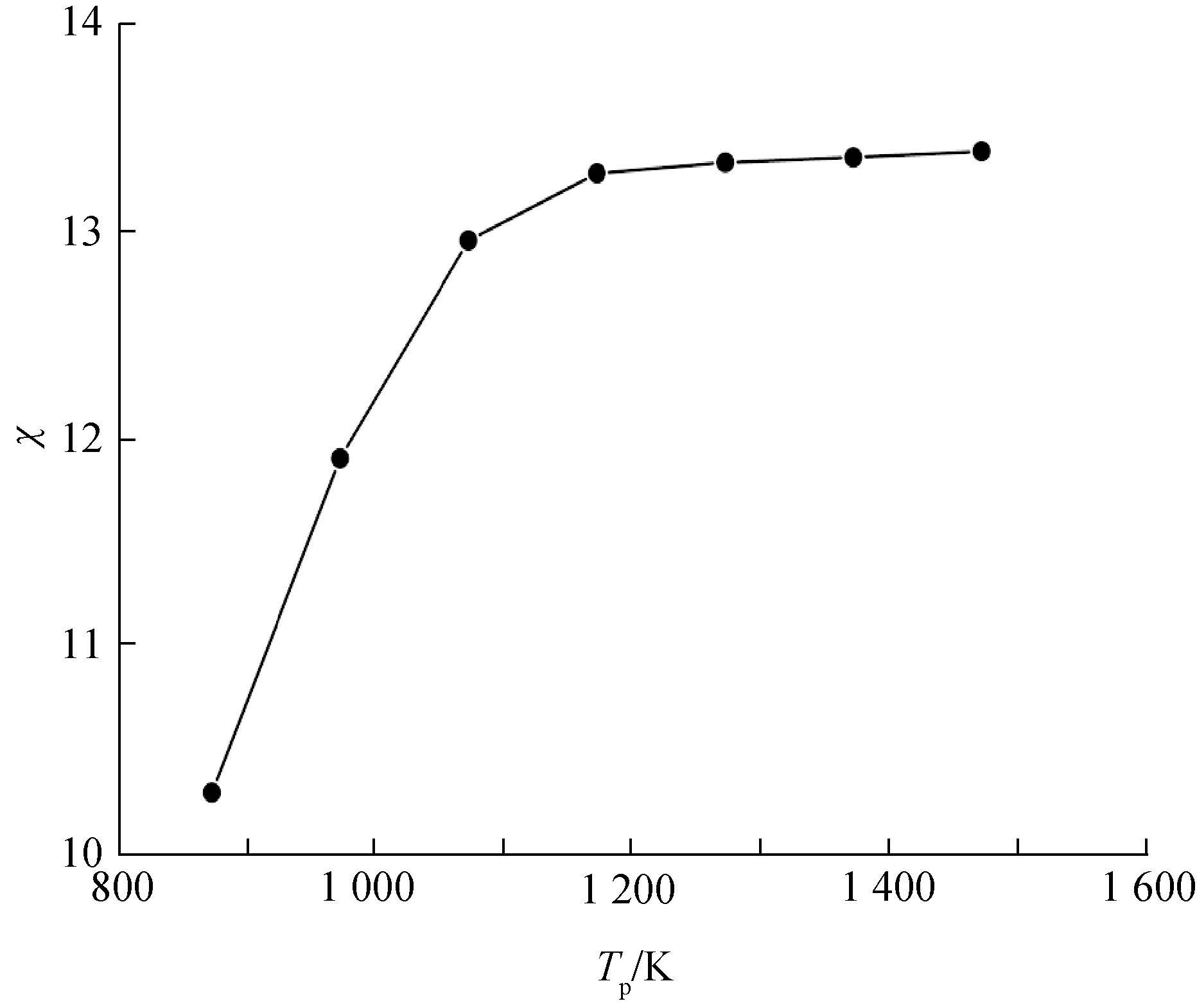图 4 不同热解终温下二次反应消耗的焦油和最终焦油的比值

2.2 加热速率对焦油生成的影响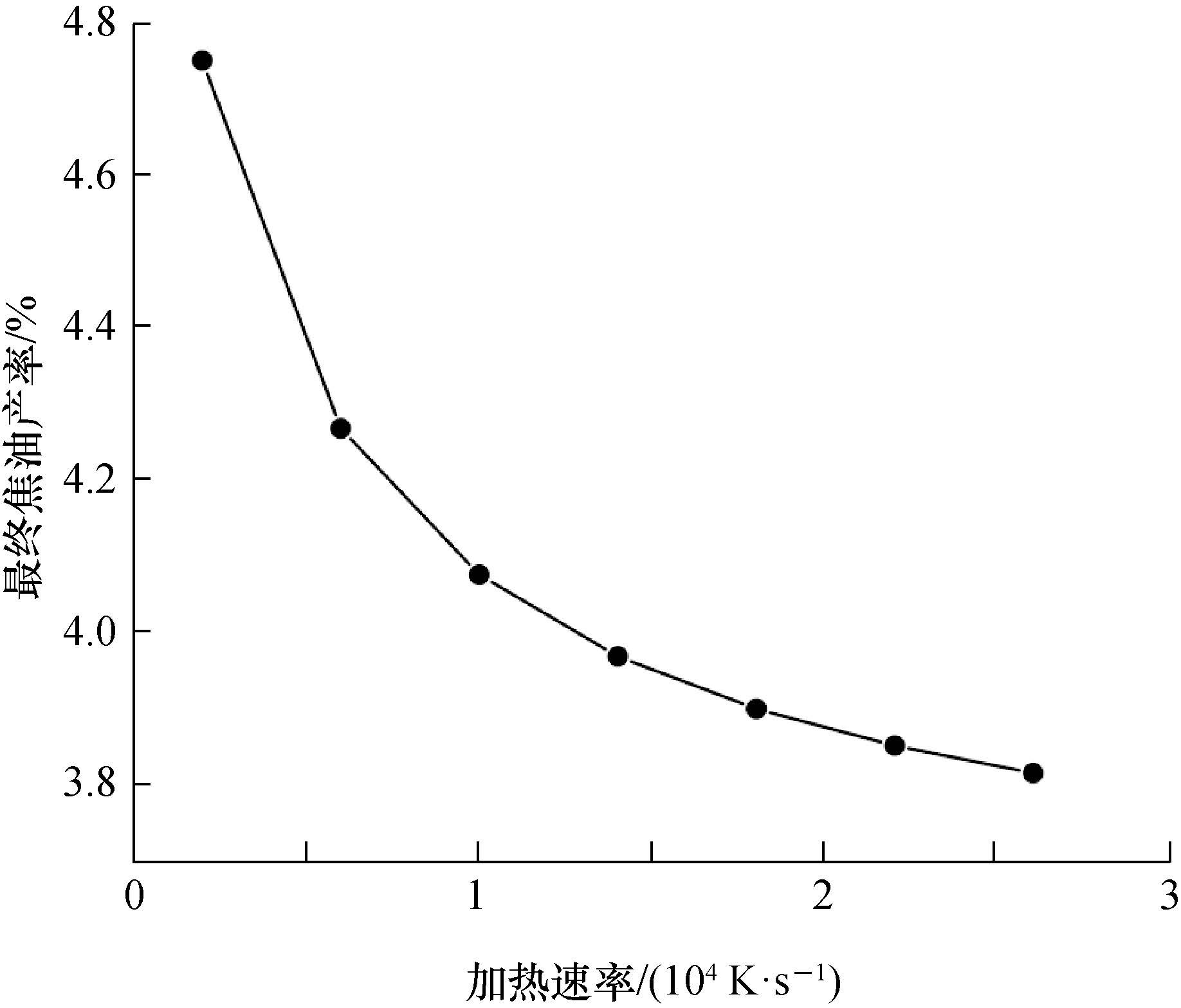图 5 最终焦油产率和加热速率的关系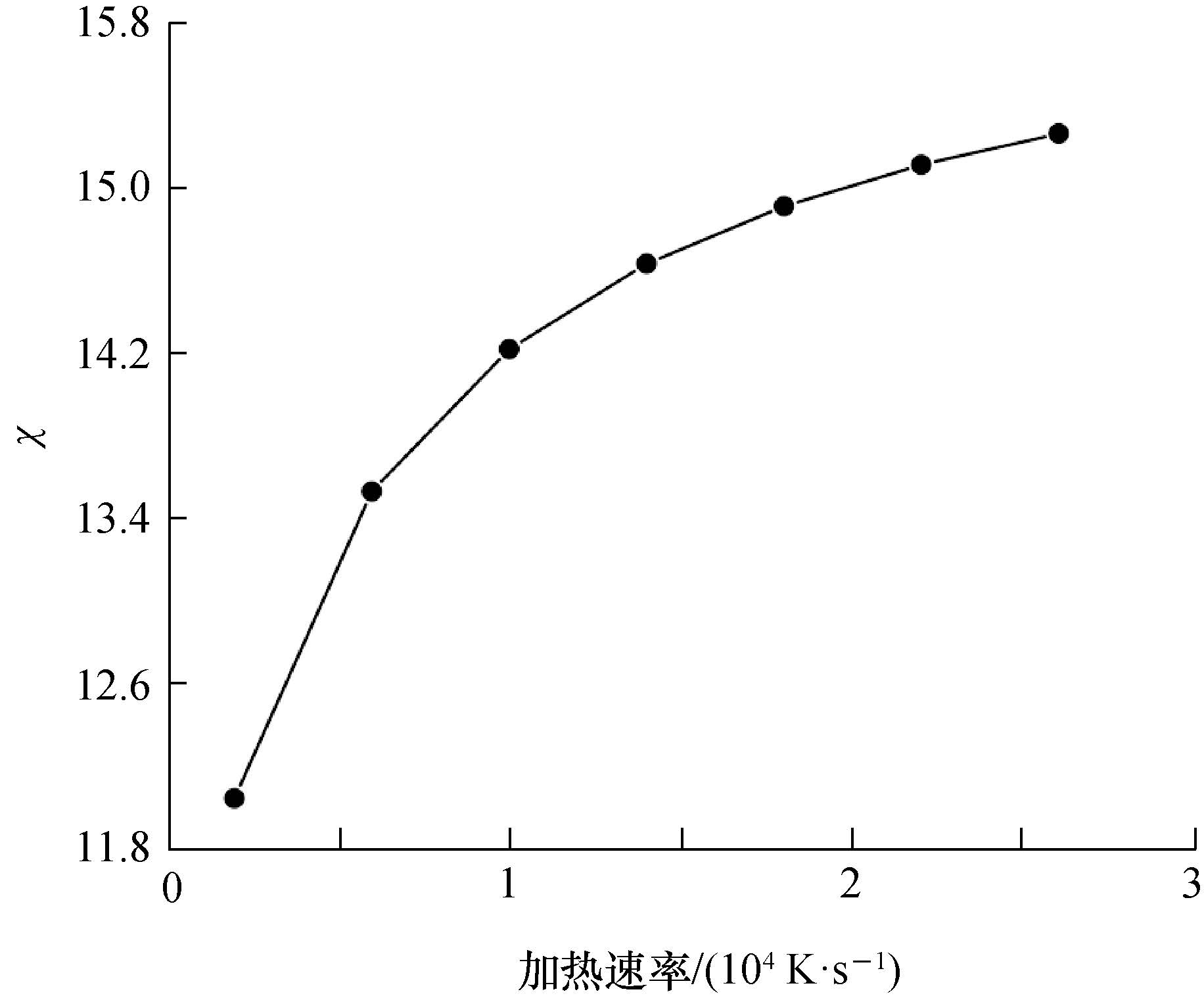图 6 不同加热速率下二次反应消耗的焦油和最终焦油的比值

2.3 颗粒粒径对焦油生成的影响图 7 最终焦油产率和颗粒粒径的关系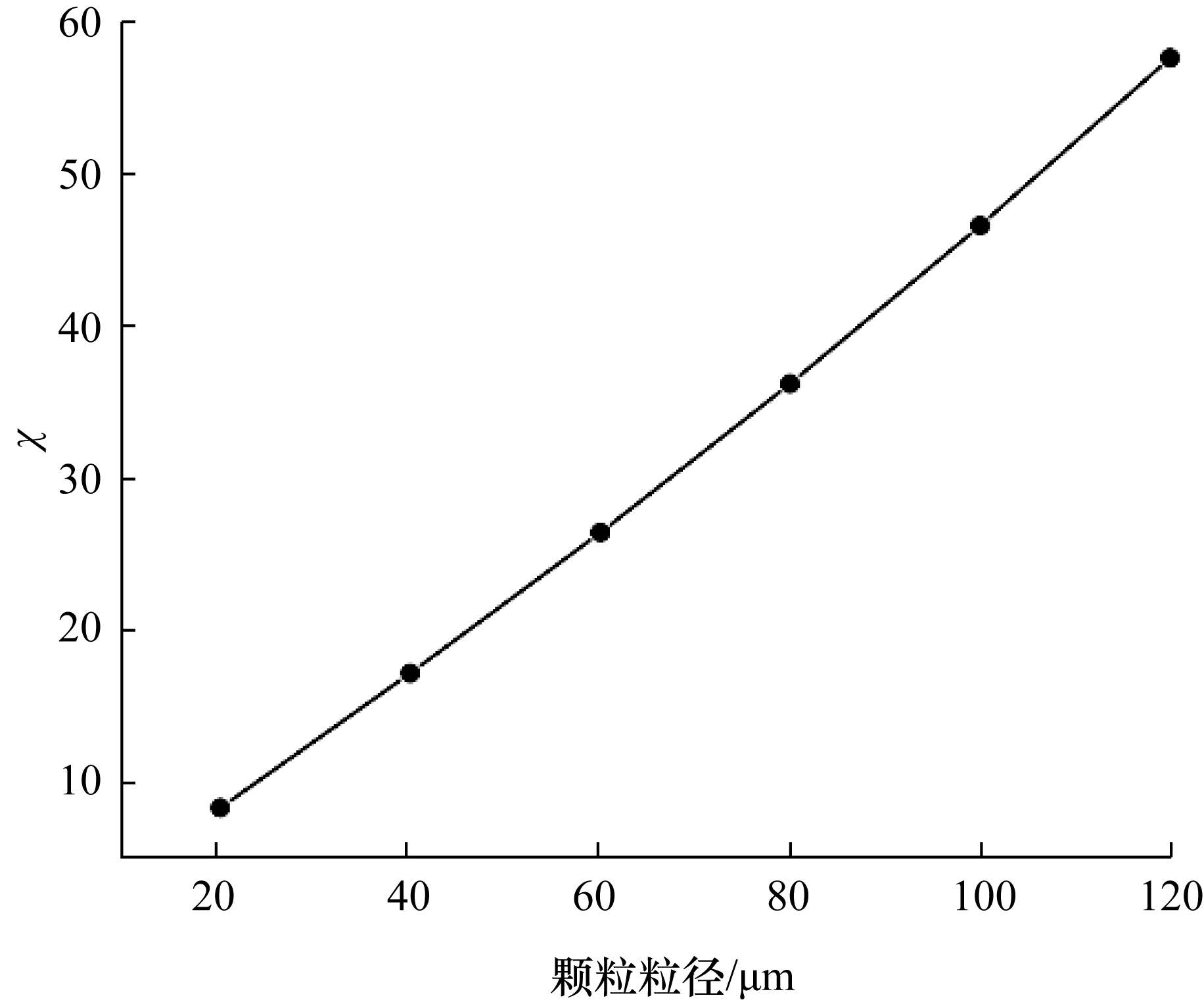图 8 不同颗粒粒径下二次反应消耗的焦油和最终焦油的比值

3 结 论

1) 热解终温和加热速率的上升会同时促进二次反应和扩散,但是对二次反应的促进更强,因此在这一对竞争性过程中更有利于二次反应,焦油的最终产率随着热解终温和加热速率的上升而下降;

2) 粒径的增大会促进二次反应,并阻碍扩散,因此相比于热解终温和加热速率,对最终焦油产率的影响更大,随着粒径的增大,二次反应增强,扩散到颗粒外部的焦油减少,焦油的最终产率下降;

3) 大部分的一次焦油都在颗粒内部发生二次反应而消耗掉,在本文计算的几种工况下,二次反应消耗的一次焦油是扩散到颗粒外部的一次焦油的10倍以上。

  王晓亮, 何榕, 陈永利. 煤颗粒热解过程中孔隙分形维数变化的数值模拟[J]. 清华大学学报: 自然科学版,2008, 48 (2) : 244 –247. WANG Xiaoliang, HE Rong, CHEN Yongli. Numerical simulation of pore fractal dimension evolution during coal pyrolysis[J]. J Tsinghua Univ : Sci & Tech,2008, 48 (2) : 244 –247. (in Chinese)  薛江涛. 流化床煤热解气化过程中焦油析出特性研究 [D]. 杭州: 浙江大学, 2005. XUE Jiangtao. Research on Tar Formation during Fluidized Bed Coal Pyrolysis and Gasification Process [D]. Hangzhou: Zhejiang University, 2005. (in Chinese)  石振晶. 煤热解焦油析出特性和深加工试验研究 [D]. 杭州: 浙江大学, 2014. SHI Zhenjing. Reasearch on Formation of Tar during Coal Pyrolysis and Deep Processing of Tar [D]. Hangzhou: Zhejiang University, 2014. (in Chinese)  Gonenc Z S, Gibbins J R, Katheklakis I E, et al. Comparison of coal pyrolysis product distributions from three captive sample techniques[J]. Fuel,1990, 69 (3) : 383 –390.  Tyler R J. Flash pyrolysis of coals: 1. Devolatilization of a Victorian brown coal in a small fluidized-bed reactor[J]. Fuel,1979, 58 (9) : 680 –686.  Heyd L E. Weight Loss Behavior of Coal during Rapid Pyrolysis and Hydropyrolysis [D]. Princeton, NJ: Princeton University, 1982.  Gibbins-Matham J, Kandiyoti R. Coal pyrolysis yields from fast and slow heating in a wire-mesh apparatus with a gas sweep[J]. Energy Fuels,1988, 2 (4) : 505 –511.  Chen Y, He R. Fragmentation and diffusion model for coal pyrolysis[J]. J Anal Appl Pyrolysis,2011, 90 (1) : 72 –79.  马亮, 何榕. 分形多孔介质中气体稳态扩散[J]. 清华大学学报: 自然科学版,2013, 53 (10) : 1459 –1463. MA Liang, HE Rong. Steady-state gas diffusion in fractal porous media[J]. J Tsinghua Univ : Sci & Tech,2013, 53 (10) : 1459 –1463. (in Chinese)  马亮, 何榕. 分形多孔介质中气体的非稳态扩散[J]. 化工学报,2013, 64 (9) : 3139 –3144. MA Liang, HE Rong. Unsteady state gas diffusion in fractal porous media[J]. CIESC Journal,2013, 64 (9) : 3139 –3144. (in Chinese)  Suuberg E M. Rapid Pyrolysis and Hydropyrolysis of Coal [D]. Boston, MA: Massachusetts Institute of Technology, 1978.  Bliek A, Van Poelje W M, Van Swaaij W, et al. Effects of intraparticle heat and mass transfer during devolatilization of a single coal particle[J]. AIChE J,1985, 31 (10) : 1666 –1681.  Wang X, He R, Chen Y. Evolution of porous fractal properties during coal devolatilization[J]. Fuel,2008, 87 (6) : 878 –884.